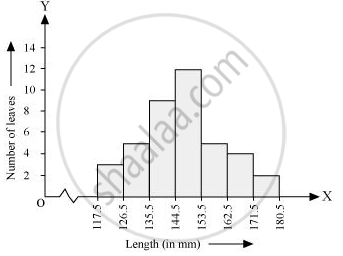Share

# The Length of 40 Leaves of a Plant Are Measured Correct to One Millimetre, and the Obtained Data is Represented in the Following Table - CBSE Class 9 - Mathematics

ConceptGraphical Representation of Data

#### Question

The length of 40 leaves of a plant are measured correct to one millimetre, and the obtained data is represented in the following table:-

 Length (in mm) Number of leaves 118 - 126 3 127 - 135 5 136 - 144 9 145 - 153 12 154 - 162 5 163 - 171 4 172 - 180 2

(i) Draw a histogram to represent the given data. [Hint: First make the class intervals continuous]

(ii) Is there any other suitable graphical representation for the same data?

(iii) Is it correct to conclude that the maximum number of leaves are 153 mm long? Why?

#### Solution

(i) It can be observed that the length of leaves is represented in a discontinuous class interval having a difference of 1 in between them. Therefore, 1/2 = 0.5 has to be added to each upper class limit and also have to subtract 0.5 from the lower class limits so as to make the class intervals continuous.

 Length (in mm) Number of leaves 117.5 − 126.5 3 126.5 − 135.5 5 135.5 − 144.5 9 144.5 − 153.5 12 153.5 − 162.5 5 162.5 − 171.5 4 171.5 − 180.5 2Taking the length of leaves on x-axis and the number of leaves on y-axis, the histogram of this information can be drawn as above.

Here, 1 unit on y-axis represents 2 leaves.

(ii) Other suitable graphical representation of this data is frequency polygon.

(iii) No, as maximum number of leaves (i.e., 12) has their length in between 144.5 mm and 153.5 mm. It is not necessary that all have their lengths as 153 mm.

Is there an error in this question or solution?

#### APPEARS IN

NCERT Solution for Mathematics Class 9 (2018 to Current)
Chapter 14: Statistics
Ex. 14.30 | Q: 4 | Page no. 259

#### Video TutorialsVIEW ALL 

Solution The Length of 40 Leaves of a Plant Are Measured Correct to One Millimetre, and the Obtained Data is Represented in the Following Table Concept: Graphical Representation of Data.
S